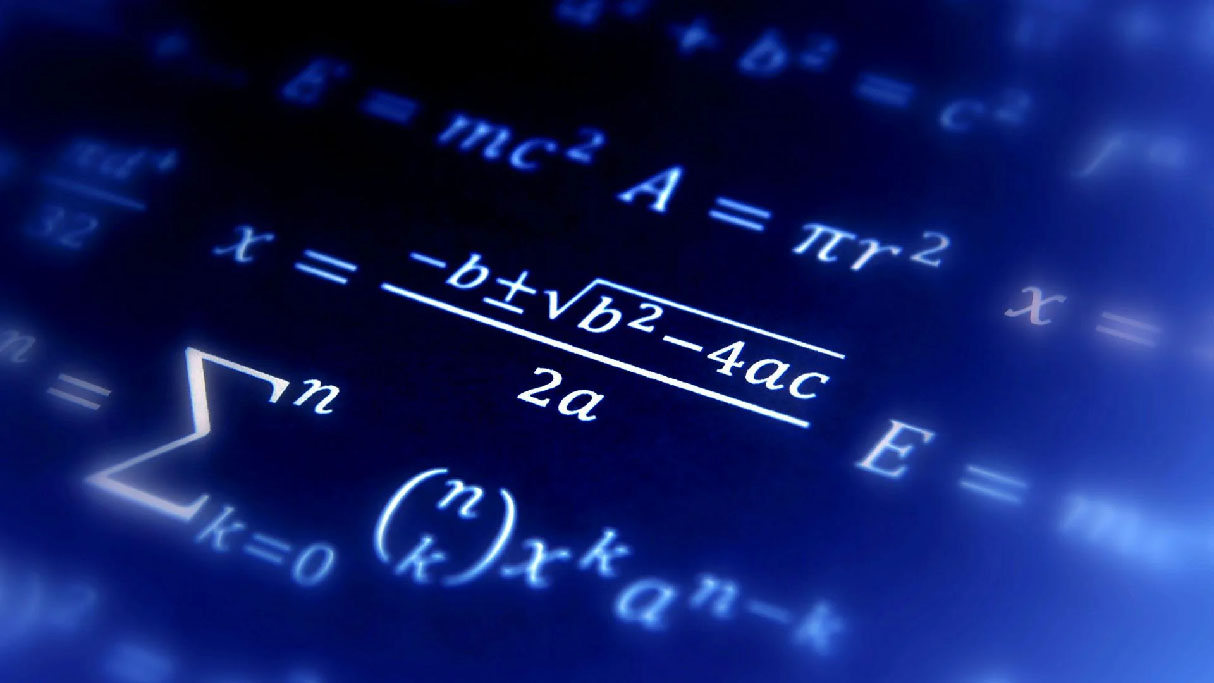# MathematicsA mathematics pre-medical course is designed to provide students with a strong foundation in mathematics and quantitative reasoning skills, which are essential for success in medical school and in the medical profession. This course covers a range of mathematical topics that are relevant to medicine, including calculus, statistics, and probability theory.

Here are some examples of topics that may be covered in a mathematics pre-medical course:

• Calculus: This course covers the fundamentals of differential and integral calculus, which are essential for understanding a wide range of topics in science and engineering.
• Statistics: This course covers the basic principles of statistical analysis, including probability theory, hypothesis testing, and regression analysis.
• Linear Algebra: This course covers the study of vectors, matrices, and linear transformations, which are essential for understanding a wide range of topics in science and engineering.
• Differential Equations: This course covers the study of differential equations, which are used to model a wide range of phenomena in the natural sciences and engineering.
• Discrete Mathematics: This course covers the study of discrete structures such as graphs and networks, which are used to model complex systems in biology and medicine.

A mathematics pre-medical course is typically offered at the undergraduate level and is a required course for students interested in applying to medical school. The course is designed to help students develop the quantitative reasoning skills needed to succeed in medical school and in the medical profession. These skills are essential for understanding and interpreting medical data, conducting research, and making informed decisions about patient care.